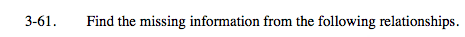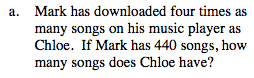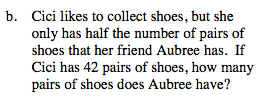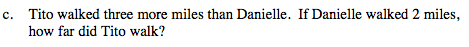### Home > MC2 > Chapter 3 > Lesson 3.2.1 > Problem3-61

3-61.Since Mark has 4 times as many songs as Chloe and he has 440 songs, what number of songs could Chloe have that would be a fourth of Mark's total songs?

Mark's songs = 4(Chloe's songs)

440 songs = 4(Chloe's songs)

What number times 4 would equal 440 songs? Use this equation to solve for Chloe's total number of songs.$\text{Cici's \# of shoes }=\frac{1}{2}(\text{Aubree's \# of shoes)}$

2(Cici's # of shoes) = Aubree's # of shoes. Since Cici has 42 pairs of shoes, use this equation to solve for the number of pairs of shoes Aubree has.

84 pairs of shoes.
Can you explain how you got this answer from the equation above?Refer to parts (a) and (b).

Tito's miles = Danielle's miles + 3 miles